# How to define square root(√) in LaTeX?

With the help of Latex, when the root symbol is used in a science document, you need to call the \sqrt command. And you have to pass two arguments in this \sqrt command, one is the value of the root and the other is the expression. Notice this syntax below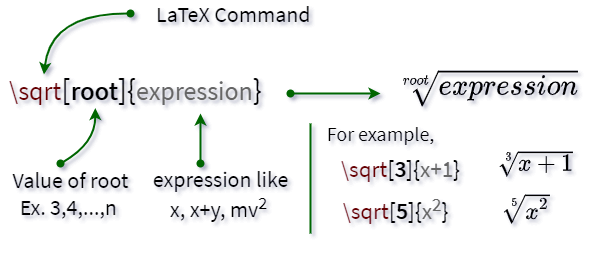However, there is no need to add root value with \sqrt command in case of square root. Then, the syntax of the command will be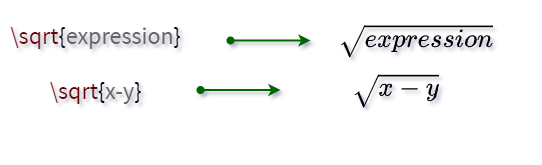For example, if we want to define the square root of x with the help of latex, then x has to be passed to the \sqrt command as an expression and there is no need to give the value of the root.

\documentclass{article}
\begin{document}
$\sqrt{x}$
\end{document}

Output :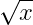And if you use nth root instead of square root then you have to pass n as the value of root.

\documentclass{article}
\begin{document}
$\sqrt[n]{x}$
\end{document}

Output :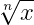## LaTeX square root Example

Below we will discuss some important concepts that you are familiar with. Because when you create a science document with latex, this concept will be useful in most cases.

### 1. Velocity from position vector in LaTeX

Here the value of velocity has to be determined using square root

\documentclass{article}
\begin{document}
$v=\sqrt{\left ( \frac{dx}{dt} \right )^{2}+\left ( \frac{dy}{dt} \right )^{2}+ \left ( \frac{dz}{dt} \right )^{2}}$
\end{document}

Output :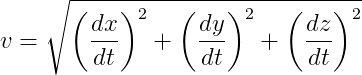Looking at the output above, you can see that the size of the square root symbol will decrease and increase dynamically according to the size of the expression.

### 2. Root of quadratic equation

You all know how to write the root of quadratic equation! Suppose, if the quadratic equation is ax²+bx+c, then root will be

\documentclass{article}
\begin{document}
$\frac{-b \pm \sqrt{b^2-4ac}}{2a}$
\end{document}


Output :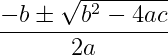### 3. Momentum from Kinetic energy

You also need to use the square root symbol when determining the momentum from kinetic energy.

\documentclass{article}
\begin{document}
$p=\sqrt{2mK}$
\end{document}

Output :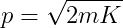### 4. Space-time equation

You all know that the Theory of Relativity has been given an important equation over time

\documentclass{article}
\begin{document}
$t'=\frac{t}{\sqrt{1-\frac{v^2}{c^2}}}$
\end{document}

Output :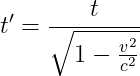Notice that the Latex \sqrt command will always return a square root symbol with dynamic size. And with this command, you just have to pass the expression.